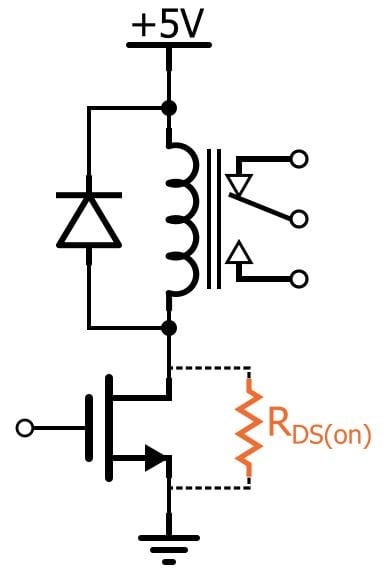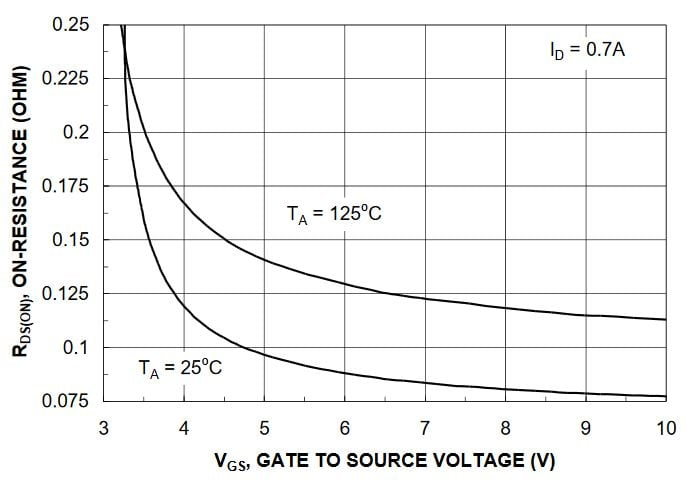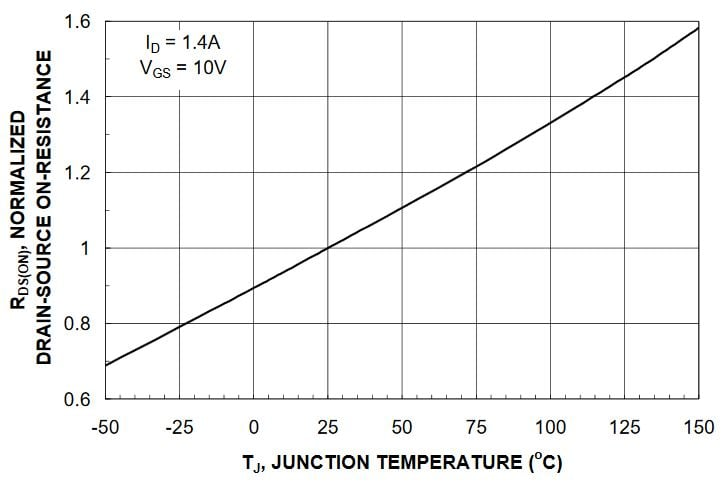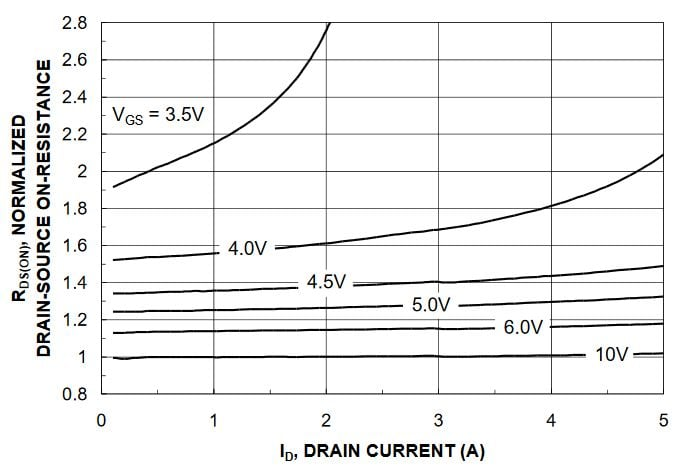Technical Article

# Understanding MOSFET On-State Drain-to-Source Resistance

September 02, 2016 by Robert Keim

## This technical brief covers some useful details regarding a common MOSFET parameter referred to as on-state resistance.

This technical brief covers some useful details regarding a common MOSFET parameter referred to as on-state resistance.

### Supporting Information

One of the most prominent specifications on datasheets for discrete MOSFETs is the drain-to-source on-state resistance, abbreviated as RDS(on). This RDS(on) idea seems so pleasantly simple: When the FET is in cutoff, the resistance between source and drain is extremely high—so high that we assume zero current flow. When the FET’s gate-to-source voltage (VGS) exceeds the threshold voltage (VTH), it is in the “on state,” and the drain and source are connected by a channel with resistance equal to RDS(on). However, if you are familiar with the actual electrical behavior of a MOSFET, you should readily recognize that this model doesn’t accord with the facts.

First, the FET does not really have an “on state.” When not in cutoff (we’re ignoring subthreshold conduction here), the FET can be in the triode region or the saturation region. Each of these regions has its own current–voltage relationship. However, we can safely assume that “on state” corresponds to the triode region because RDS(on) is relevant in the context of switch circuits, not small-signal amplifiers, and switch circuits—e.g., for driving a motor or controlling a relay—employ the cutoff and triode regions.But still, the triode region is governed not by a mere resistance but by a rather complicated equation:

$$I_D=\mu_nC_{ox}\frac{W}{L}\left(\left(V_{GS}-V_{TH}\right)V_{DS}-\frac{1}{2}V_{DS}^2\right)$$

(This is for an NMOS device; a PMOS device would have µp instead of µn.) However, if we ignore the VDS2 term, the equation can be simplified as follows:

$$I_D=\mu_nC_{ox}\frac{W}{L}\left(V_{GS}-V_{TH}\right)V_{DS}$$

Now we do indeed have a linear (i.e., resistive) relationship between drain-to-source current (ID) and drain-to-source voltage (VDS). However, the “resistance” is not constant, as in the case of a mere resistor; rather, the resistance corresponds to

$$\frac{1}{\mu_nC_{ox}\frac{W}{L}\left(V_{GS}-V_{TH}\right)}$$

This brings us to an important point about RDS(on): it is influenced by the gate-to-source voltage. Here’s an example taken from the datasheet for Fairchild’s NDS351AN MOSFET:The typical threshold voltage for this part is given as 2.1 V. If you look quickly at the VTH spec and very quickly at the RDS(on) spec, you might think that you can drive this FET with a 3.3 V logic signal and achieve the advertised on-state-resistance performance. This would be a bit careless considering that the datasheet clearly specifies the gate-to-source voltage that corresponds to the RDS(on) spec; however, one or two RDS(on)/VGS data points do not convey the extreme increase in on-state resistance that applies to gate-to-source voltages that are actually well above the typical VTH. So the morals of the story are 1) remember that on-state (i.e., triode-region) resistance is dependent on VGS and 2) for detailed information refer to the plot of RDS(on) vs. VGS.

Furthermore, on-state resistance is not equal to the resistance expressed by the triode-region equation given above. The latter is the resistance of the MOSFET’s channel, whereas on-state resistance encompasses other sources of resistance—bond wires, the epitaxial layer, etc. Resistance characteristics are influenced by manufacturing technology, and the respective contributions of the different components of RDS(on) vary according to the voltage range for which a particular device is intended.

Two additional factors that affect on-state resistance are junction temperature and drain current, as shown in these two plots from the NDS351AN datasheet:Thus, you may need to shop around and spend some quality time with a few datasheets before you find the right MOSFET for a particular switch application.• R
Ritalie September 05, 2016

This was absolutely perfect. It was very easy to understand and gave me a better understanding.

Like.
• M
murras1 September 23, 2016

It would be helpful if this article included the VI characteristics curve of the device under discussion to clarify the definitions of cutoff vs triode vs saturation regions. The CMOS saturation region is defined differently than in bipolar devices - which is often confused in literature and in design documentation.

Like.
•bramlabs November 29, 2016

This is why i really love allaboutcircuit. They explain it with an easy way for people to understand it !

Like.